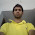# Calculate Distance of Two Points using C Structure

Let A (x1, y1) and B (x2, y2) are two points. Then the formula to calculate the distance between these two points is:
In general, we can take 4 variables x1, y1, x2, y2 and calculate their distance using above formula. But it will be smarter if we use a structure that represent a single point. Here is a example program:

```#include <stdio.h>
#include <stdlib.h>
#include <math.h>

struct Point {
int x, y;
};

double getDistance(struct Point a, struct Point b)
{
double distance;
distance = sqrt((a.x - b.x) * (a.x - b.x) + (a.y-b.y) *(a.y-b.y));
return distance;
}

int main()
{
struct Point a, b;
printf("Enter coordinate of point a: ");
scanf("%d %d", &a.x, &a.y);
printf("Enter coordinate of point b: ");
scanf("%d %d", &b.x, &b.y);
printf("Distance between a and b: %lf\n", getDistance(a, b));

return 0;
}
```
Here is sample run of the program:

Share:

#### 1 comments :

1.Spam comments will be deleted. :)